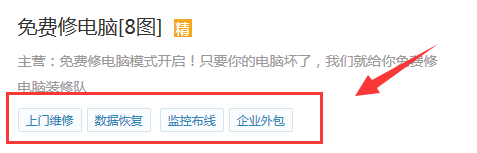[field:字段 runphp=yes]
\$arr = explode(',',@me);
\$result = '';
for(\$index=0;\$index<count(\$arr);\$index++){
\$result .= "<span class='tag'>\$arr[\$index]</span>";
}
@me = \$result;
[/field:字段]

{dede:field.字段 runphp=yes}
\$arr = explode(',',@me);
\$result = '';
for(\$index=0;\$index<count(\$arr);\$index++){
\$result .= "<span class='tag'>\$arr[\$index]</span>";
}
@me = \$result;
{/dede:field.字段}